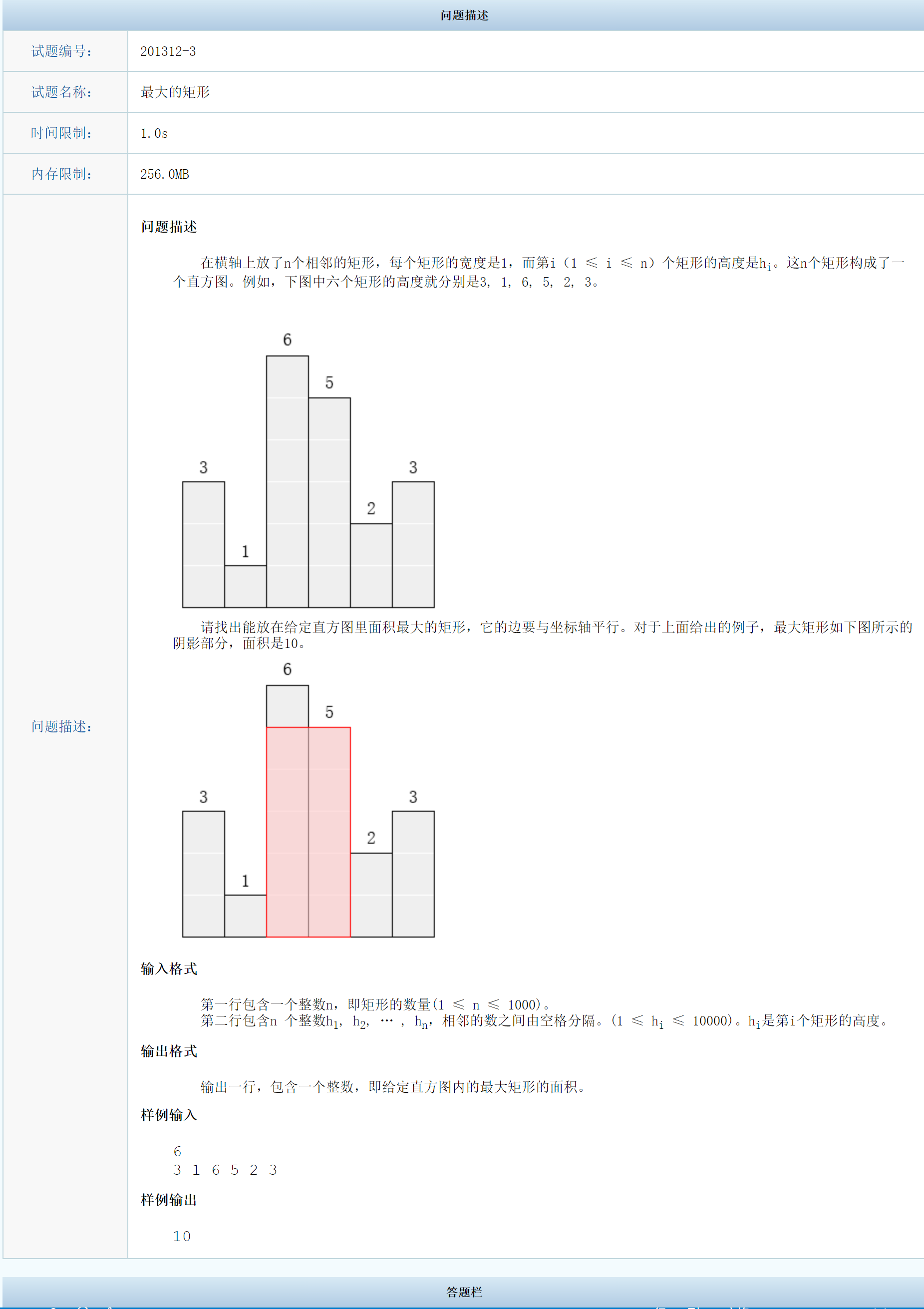# 201312-3最大的矩形

## 题目## 代码

  1 2 3 4 5 6 7 8 9 10 11 12 13 14 15 16 17 18 19 20 21 22 23 24 25  /* 最大的矩形 */ #include using namespace std; int n; int h[1000 + 10], d[1000 + 10]; int main() { cin >> n; for (int i = 0; i < n; i++) { cin >> h[i]; } int maxn = 0; for (int i = 0; i < n; i++) { int j = i, k = 1; // k用于表示以h[i]为高的最大矩形的宽 while (j - 1 >= 0 && h[j - 1] > h[i]) { k++, j--; } j = i; while (j + 1 < n && h[j + 1] > h[i]) { k++, j++; } maxn = max(maxn, h[i] * k); } cout << maxn; return 0; }